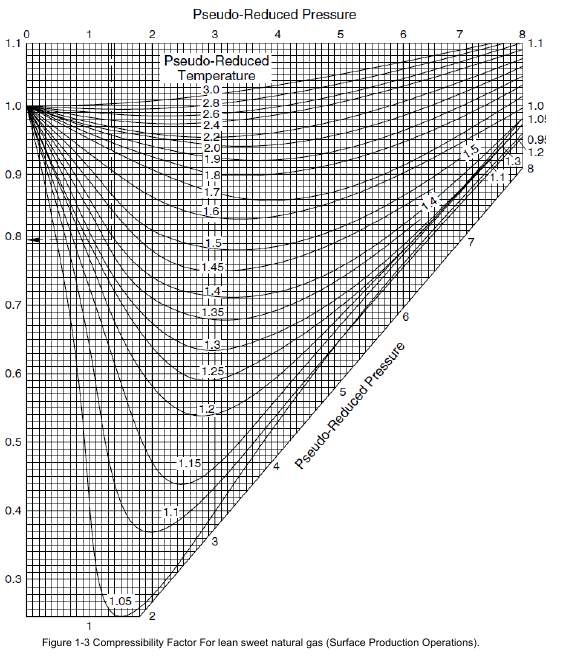# Compressibility Factor (z)

The Compressibility factor, Z is a dimensionless parameter less than 1.00 that represents the deviation of
a real gas from an ideal gas. Hence it is also referred to as the gas deviation factor. At low pressures and
temperatures Z is nearly equal to 1.00 whereas at higher pressures and temperatures it may range
between 0.75 and 0.90. The actual value of Z at any temperature and pressure must be calculated taking
into account the composition of the gas and its critical temperature and pressure. Several graphical and
analytical methods are available to calculate Z. Among these, the Standing-Katz, and CNGA (California
natural gas association) methods are quite popular. The critical temperature and the critical pressure of a
gas are important parameters that affect the compressibility factor and are defined as follows.
The critical temperature of a pure gas is that temperature above which the gas cannot be compressed
into a liquid, however much the pressure. The critical pressure is the minimum pressure required at the
critical temperature of the gas to compress it into a liquid.
As an example, consider pure methane gas with a critical temperature of 343 0R and critical pressure of
666 psia (Table 1-1).

The reduced temperature of a gas is defined as the ratio of the gas temperature to its critical temperature,
both being expressed in absolute units (0R). It is therefore a dimensionless number.
Similarly, the reduced pressure is a dimensionless number defined as the ratio of the absolute pressure
of gas to its critical pressure.
Therefore we can state the following: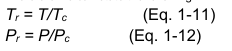Where
P = pressure of gas, psia
T = temperature of gas, 0R
Tr = reduced temperature, dimensionless
Pr = reduced pressure, dimensionless
Tc = critical temperature, 0R
Pc = critical pressure, psia
Example1-4: Using the preceding equations, the reduced temperature and reduced pressure of a sample
of methane gas at 70 0F and 1200 psia pressure can be calculated as follows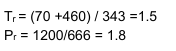For natural gas mixtures, the terms pseudo-critical temperature and pseudo-critical pressure are used.
The calculation methodology will be explained shortly. Similarly we can calculate the pseudo-reduced
temperature and pseudo-reduced pressure of a natural gas mixture, knowing its pseudo-critical
temperature and pseudo-critical pressure.
The Standing-Katz chart, Fig. 1.3 can be used to determine the compressibility factor of a gas at any
temperature and pressure, once the reduced pressure and temperature are calculated knowing the
critical properties.
Pseudo-critical properties allow one to evaluate gas mixtures. Equations (1-13) and (1-14) can be used to
calculate the pseudo-critical properties for gas mixtures: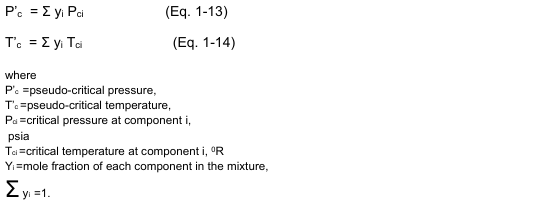Example 1-5: Calculate the Compressibility factor for the following Gas mixture at 1000F and 800 psig: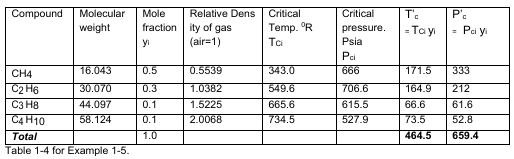Using Equation 1-11 and 1-12
T` r = (100+460)/464.5
=1.2
P`r = (800+14.7)/659.4 = 1.23
From fig.1-3. Compressibility factor is approximately, z= 0.72
Calculating the compressibility factor for example 1-4, of the gas at 70 0F and 1200 psia, using Standing-
Katz chart, fig. 1-3. Z = 0.83 approximately. For ) Tr = 1.5 , Pr = 1.8).
Another analytical method of calculating the compressibility factor of a gas is using the CNGA equation as
follows: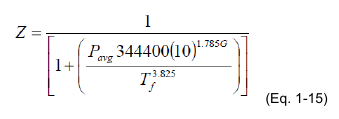Where
Pavg = Gas pressure, psig. [psig = (psia – 14.7)]
Tf
= Gas temperature, 0R
G = Gas gravity (air = 1.00)
The CNGA equation for compressibility factor is valid when the average gas pressure Pavg is greater than
100 psig. For pressures less than 100 psig, compressibility factor is taken as 1.00. It must be noted that
the pressure used in the CNGA equation is the gauge pressure, not the absolute pressure.
Example 1-6: Calculate the compressibility factor of a sample of natural gas (gravity = 0.6) at 80 0F and
1000 psig using the CNGA equation.
Solution:
From the Eq. (1.15), the compressibility factor is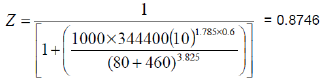The CNGA method of calculating the compressibility, though approximate, is accurate enough for most
gas pipeline hydraulics work and process calculations.